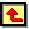# Appendix III. XPS Data

This Appendix presents X-ray photoelectron spectra relevant to the results presented within Chapters 6 and 7.  The appendix is split into two sections, which contain data for films grown at various H2S additions to (a) 1%CH4/H2 and 0.5%CS2/H2 gas mixtures and (b) 51%CH4/49%CO2 gas mixtures

## (a). H2S/1%CH4/H2 and 0.5%CS2/H2 Gas Mixtures

All the following data is for diamond films deposited on undoped Si (100) wafers under the conditions outlined below (see Chapter 6).

 Gas Mixture H2S/1%CH4/H2 or 0.5%CS2/H2 (S14) Total Flow rate / sccm 200 H2S Addition Varies between films Applied Microwave Power / W 1000 Chamber Pressure / Torr 40 Substrate Temperature / °C 900

The sample code, gas phase H2S addition and S/C ratio for each film is given.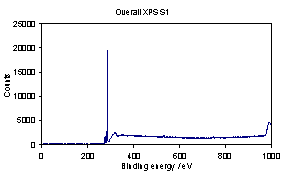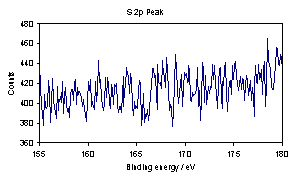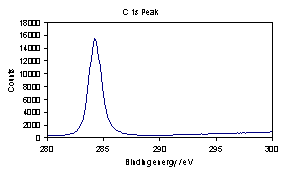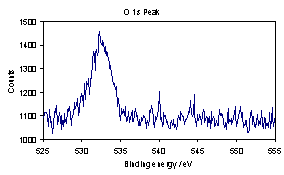(Sample S1, 100 ppm H2S addition, film S/C ratio = 0.00 %)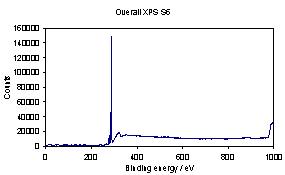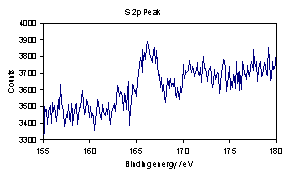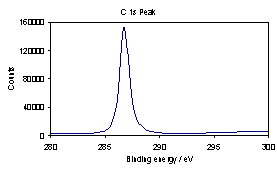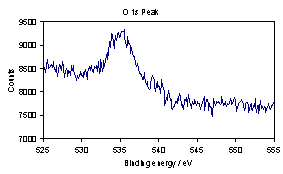(Sample S6, 1000 ppm H2S addition, film S/C ratio = 0.026 %)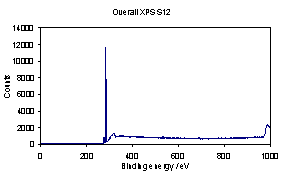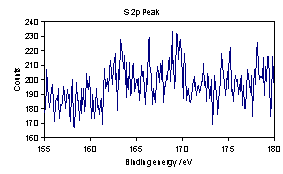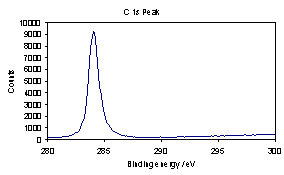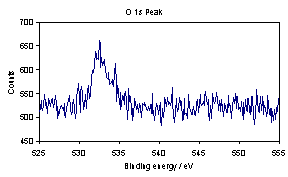(Sample S12, 3000 ppm H2S addition, film S/C ratio = 0.11 %)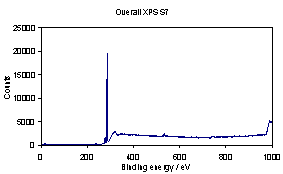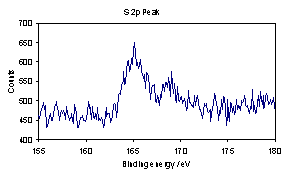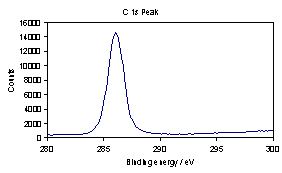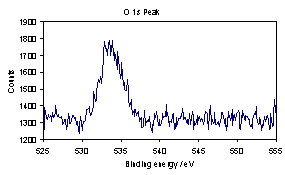(Sample S7, 5000 ppm H2S addition, film S/C ratio = 0.22 %)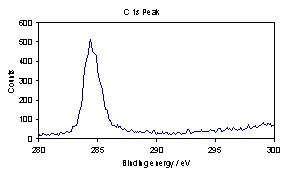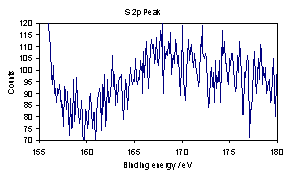(Sample S7 REPEAT, 5000 ppm H2S addition, film S/C ratio = 0.44 %)

Sample S7 was then Ar etched for ~ 2 hours (etch rate not known).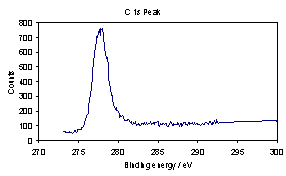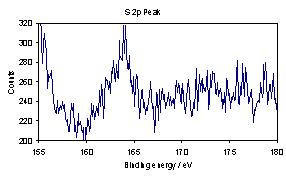(Sample S7 AFTER ETCH, 5000 ppm H2S addition, film S/C ratio = 0.34 %)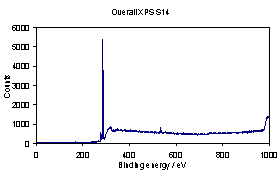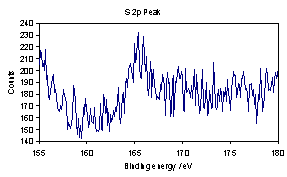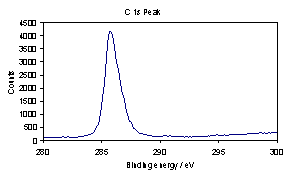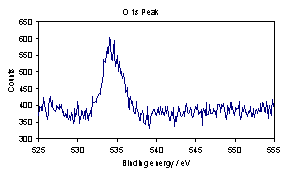(Sample S14, 0.5%CS2/H2, film S/C ratio = 0.16 %)

## (b). H2S/51%CH4/49%CO2 Gas Mixtures

All the following data is for diamond films deposited on undoped Si (100) wafers under the conditions outlined below (see Chapter 7).

 Gas Mixture H2S/51%CH4/49%CO2 Total Flow rate / sccm 100 H2S Addition Varies between films Applied Microwave Power / W 1000 Chamber Pressure / Torr 40 Substrate Temperature / °C 900

The sample code, Tsub, gas phase H2S addition and S/C ratio for each film is given.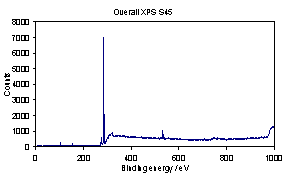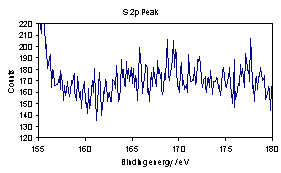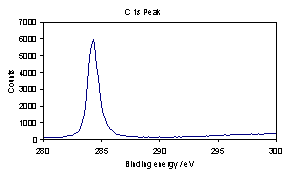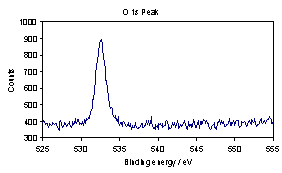(Sample S45, Tsub = 620°C, 2000 ppm H2S addition, film S/C ratio = 0.00 %)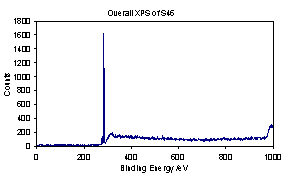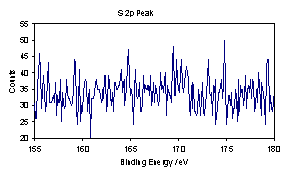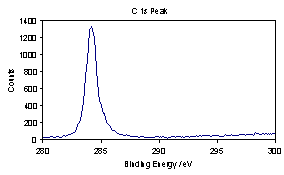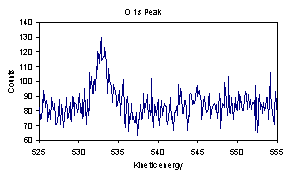(Sample S46, Tsub = 620°C, 3500 ppm H2S addition, film S/C ratio = 0.00 %)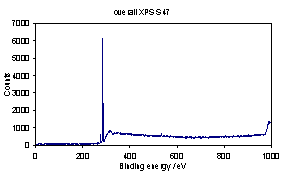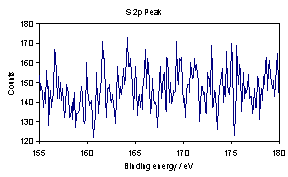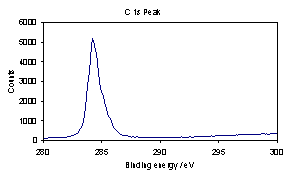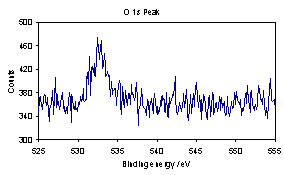(Sample S47, Tsub = 620°C, 5000 ppm H2S addition, film S/C ratio = 0.00 %)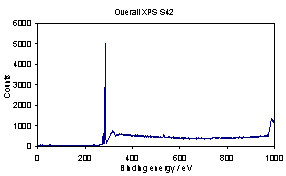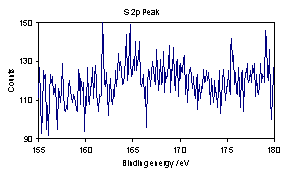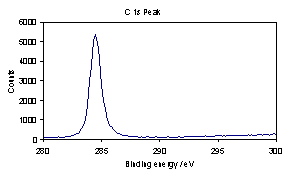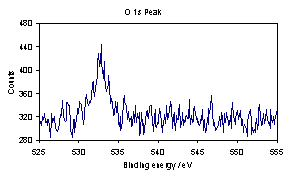(Sample S42, Tsub = 900°C, 2000 ppm H2S addition, film S/C ratio < 0.02 %)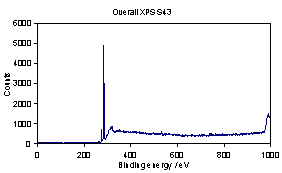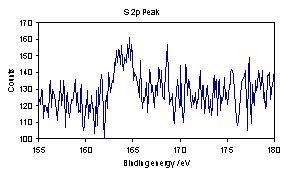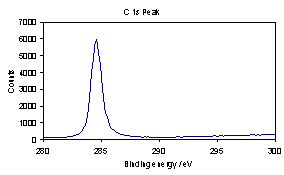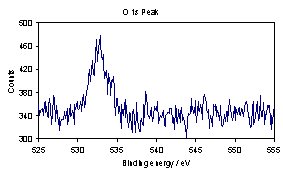(Sample S43, Tsub = 900°C, 3500 ppm H2S addition, film S/C ratio = 0.07 %)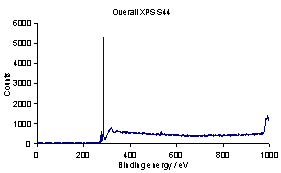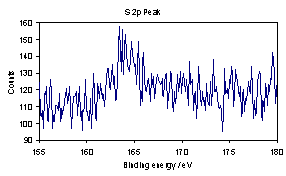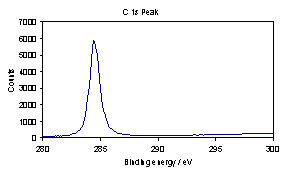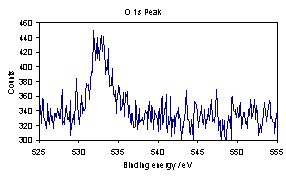(Sample S44, Tsub = 900°C, 5000 ppm H2S addition, film S/C ratio = 0.08 %)